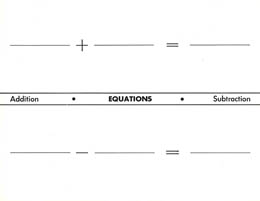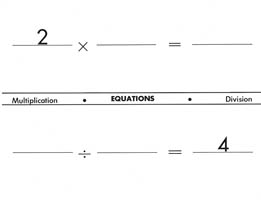Home
 Equations Game Materials: Deck of Fraction Bars and 4 sheets of paper per group plus pencils Equations mats can also be downloaded.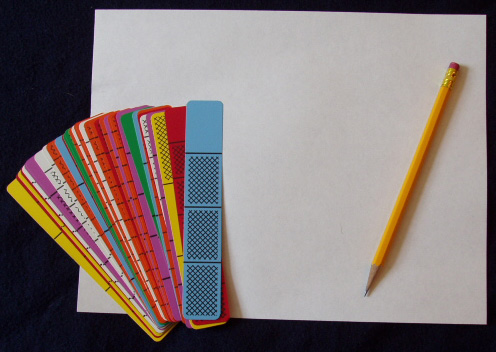Ask each group to select 10 random bars, two of each color, and try to form four equations: one for addition, one for subtraction, one for multiplication, and one for division. For the multiplication and division equations they can use any whole numbers except zero. For example, the numbers 3 and 4 were used in forming the equations below. If the bars are placed on sheets of paper, the operation signs, equality signs, and whole numbers for the products and quotients can be written on the sheets. The following example shows that all four equations were formed by using the 10 bars selected, but this is not always possible.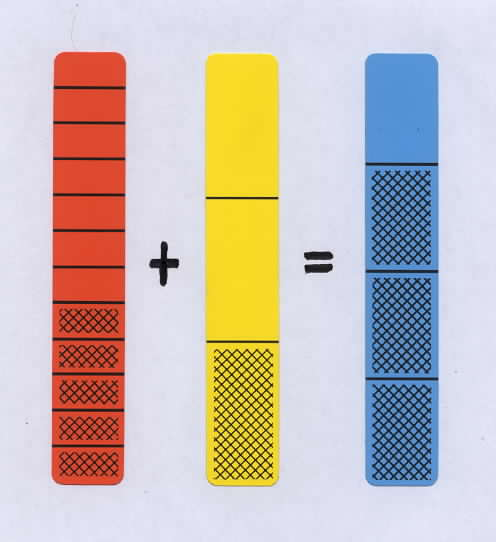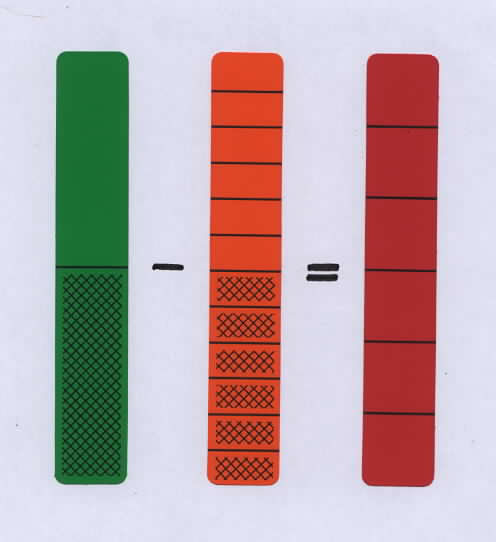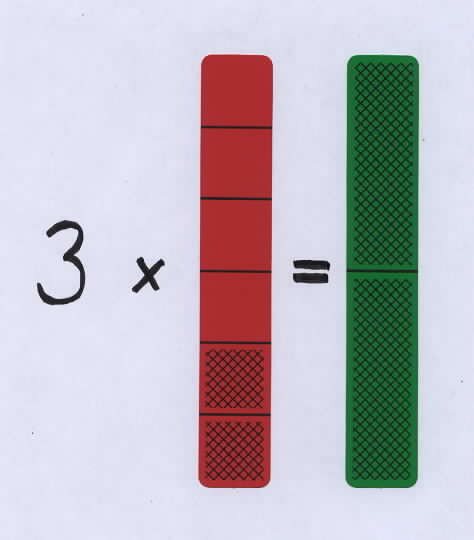Each bar that is used in an equation counts as one point. Ask if any groups were able to form four equations, that is, to score 10 points. Sometimes when equations for all four different operations cannot be formed, players like to continue by selecting an additional bar or two to see if they can form more equations. Example of Student Writing Projects The following example of a fifth grade student’s writing about the Equations Game shows the student has learning disabilities, but by using Fraction Bars was able to understand forming addition and subtraction equations.This student below has formed an addition equation with the bars for 1/12, 11/12, and 4/4. Now he is trying to form a subtraction equation that will include the whole bar in his right hand.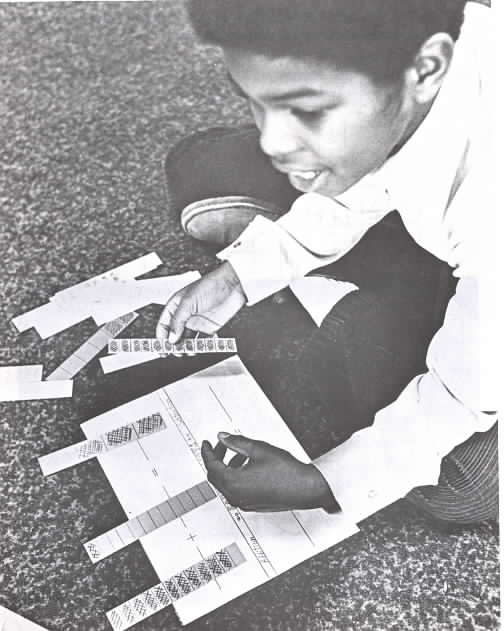Photo courtesy of Herb Moyer
 Here are examples of two equation mats for the four fraction operations. Eleven of these mats can be run off for class activities.